# Grassmann manifold

(diff) ← Older revision | Latest revision (diff) | Newer revision → (diff)

Grassmannian

The set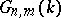,, of all-dimensional subspaces in an-dimensional vector spaceover a skew-field. Ifis a field, thencan be imbedded in a-dimensional projective space overas a compact algebraic variety with the aid of Grassmann coordinates (cf. Exterior algebra). In the study of the geometrical properties of a Grassmann manifold an important role is played by the so-called Schubert varieties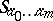,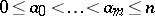, which are defined as follows. If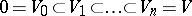is a flag of subspaces, i.e. a chain of subspaces such that also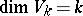, then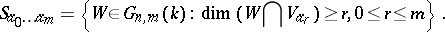Any-dimensional algebraic subvariety in a Grassmann manifoldis equivalent to a unique integer combination of the varieties, where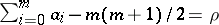(see ).

Ifis the fieldof real numbers, the fieldof complex numbers or the skew-field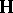of quaternions, a Grassmann manifold overcan be regarded as a compact analytic manifold (which is real iforand complex if). These manifolds are distinguished by the fact that they are the classifying spaces for the classical groups (cf. Classical group)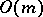,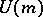and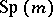, respectively. More exactly, for any CW-complexof dimension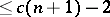, where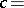1, 2 and 4, respectively, the set of isomorphism classes of-dimensional vector bundles overwith baseis in a natural one-to-one correspondence with the set of homotopy classes of continuous mappings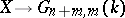. A similar theory concerning the groups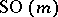and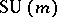leads to the study of the Grassmann manifold(or) of oriented-dimensional spaces in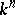. The manifolds listed above are closely connected, in particular, with the theory of characteristic classes (cf. Characteristic class).

The role played by Grassmann manifolds in topology necessitated a detailed study of their topological invariants. The oldest method of this study was based on Schubert varieties, with the aid of which a cell decomposition for() is readily constructed. It is found, in particular, that the cyclesform a basis of the homology groups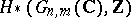,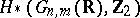,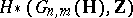. Cohomology algebras of Grassmann manifolds and the effect of Steenrod powers on them have also been thoroughly studied .

Another aspect of the theory of Grassmann manifolds is that they are homogeneous spaces of linear groups over the corresponding skew-field, and represent basic examples of irreducible symmetric spaces (cf. Symmetric space).

Manifolds which are analogous to Grassmann manifolds can also be constructed from subspaces of infinite-dimensional vector spaces. In particular, an important role in the theory of deformation of analytic structures is played by a Banach analytic manifold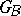, the elements of which are the closed subspaces of a Banach spaceoverwith a closed direct complement.

How to Cite This Entry:
Grassmann manifold. Encyclopedia of Mathematics. URL: http://encyclopediaofmath.org/index.php?title=Grassmann_manifold&oldid=11701
This article was adapted from an original article by A.L. Onishchik (originator), which appeared in Encyclopedia of Mathematics - ISBN 1402006098. See original article